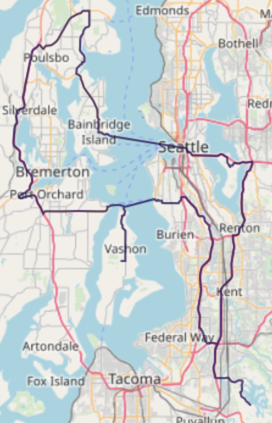# Multiple Routing on Seattle Road Network

Solve graph with multiple destinations using a road network

The following is a complete example, using the Python API, of solving a graph created with Seattle road network data for a multiple routing problem via the /solve/graph endpoint. For more information on Network Graphs & Solvers, see Network Graphs & Solvers Concepts.

## Prerequisites

The prerequisites for running the multiple routing solve graph example are listed below:

### Python API Installation

The native Kinetica Python API is accessible through the following means:

#### PyPI

The Python package manager, pip, is required to install the API from PyPI.

1. Install the API:

 ``````1 `````` ``````pip install gpudb --upgrade ``````
2. Test the installation:

 ``````1 `````` ``````python -c "import gpudb;print('Import Successful')" ``````

If Import Successful is displayed, the API has been installed as is ready for use.

#### Git

1. In the desired directory, run the following, but be sure to replace <kinetica-version> with the name of the installed Kinetica version, e.g., v7.1:

 ``````1 `````` ``````git clone -b release/ --single-branch https://github.com/kineticadb/kinetica-api-python.git ``````

 ``````1 `````` ``````cd kinetica-api-python ``````
3. In the root directory of the unzipped repository, install the Kinetica API:

 ``````1 `````` ``````sudo python setup.py install ``````
4. Test the installation (Python 2.7 (or greater) is necessary for running the API example):

 ``````1 `````` ``````python examples/example.py ``````

### Data File

The example script references the `road_weights.csv` data file, mentioned in the Prerequisites, in the current local directory, by default. This directory can specified as a parameter when running the example script.

## Script Detail

This example is going to demonstrate solving for the shortest possible route between destination points and a source point located in a Seattle road network.

### Constants

Several constants are defined at the beginning of the script:

• SCHEMA -- the name of the schema in which the tables supporting the graph creation and match operations will be created

Important

The schema is created during the table setup portion of the script because the schema must exist prior to creating the tables that will later support the graph creation and match operations.

• TABLE_SRN -- the name of the table into which the Seattle road network dataset is loaded

• GRAPH_S -- the Seattle road network graph

• TABLE_GRAPH_S_MRSOLVED -- the solved Seattle road network graph using the MULTIPLE_ROUTING solver type

Constant Definitions
 ``````1 2 3 4 5 `````` ``````SCHEMA = "tutorial_graph" TABLE_SRN = SCHEMA + ".seattle_road_network" GRAPH_S = SCHEMA + ".seattle_road_network_graph" TABLE_GRAPH_S_MRSOLVED = GRAPH_S + "_multiple_routing_solved" ``````

### Graph Creation

One graph is used for the multiple routing solve graph example utilized in the script: seattle_road_network_graph, a graph based on the Seattle road network dataset (the CSV file mentioned in Prerequisites).

The seattle_road_network_graph graph is created with the following characteristics:

• It is directed because the roads in the graph have directionality (one-way and two-way roads)
• It has no explicitly defined nodes because the example relies on implicit nodes attached to the defined edges
• The edges are identified by WKTLINE, using WKT LINESTRINGs from the WKTLINE column of the seattle_road_network table. The road segments' directionality (DIRECTION) is derived from the TwoWay column of the seattle_road_network table.
• The weights (VALUESPECIFIED) are represented using the time taken to travel the segment found in the time column of the seattle_road_network table. The weights are matched to the edges using the same WKTLINE column as edges (EDGE_WKTLINE) and the same TwoWay column as the edge direction (EDGE_DIRECTION).
• It has no inherent restrictions for any of the nodes or edges in the graph
• It will be replaced with this instance of the graph if a graph of the same name exists (recreate)
 `````` 1 2 3 4 5 6 7 8 9 10 11 12 13 14 15 16 17 18 `````` ``````create_s_graph_response = kinetica.create_graph( graph_name = GRAPH_S, directed_graph = True, nodes = [], edges = [ TABLE_SRN + ".WKTLINE AS WKTLINE", TABLE_SRN + ".TwoWay AS DIRECTION" ], weights = [ TABLE_SRN + ".WKTLINE AS EDGE_WKTLINE", TABLE_SRN + ".TwoWay AS EDGE_DIRECTION", TABLE_SRN + ".time AS VALUESPECIFIED" ], restrictions = [], options = { "recreate": "true" } ) ``````

### Multiple Routing

Before the seattle_road_network_graph graph is solved, the source node and destination nodes are defined.

Define Source & Destination Locations
 ``````1 2 3 4 5 6 7 `````` ``````source_node = "POINT(-122.1792501 47.2113606)" destination_nodes = [ "POINT(-122.2221 47.5707)", "POINT(-122.541017 47.809121)", "POINT(-122.520440 47.624725)", "POINT(-122.467915 47.427280)" ] ``````

Next, the graph is solved with the solve results being exported to the response:

Solve Graph
 ``````1 2 3 4 5 6 7 8 `````` ``````solve_s_mrgraph_response = kinetica.solve_graph( graph_name = GRAPH_S, solver_type = "MULTIPLE_ROUTING", source_nodes = [source_node], destination_nodes = destination_nodes, solution_table = TABLE_GRAPH_S_MRSOLVED, options = {"export_solve_results": "true"} )["result_per_destination_node"] ``````

The cost for the source node to visit the destination nodes is represented as time in minutes:

Solve Graph Results
 ``````1 2 `````` ``````Cost (in minutes) for source node ID POINT(-122.1792501 47.2113606) to visit destination node IDs ['POINT(-122.2221 47.5707)', 'POINT(-122.541017 47.809121)', 'POINT(-122.520440 47.624725)', 'POINT(-122.467915 47.427280)']: 240.35945638020834 ``````

The solution output to WMS:• the `solve_graph_seattle_multi_route.py` script is in the current directory
• the `road_weights.csv` file is in the current directory or use the data_dir parameter to specify the local directory containing it
 ``````1 `````` ``````python solve_graph_seattle_multi_route.py [--url ] --username --password [--data_dir ] ``````